# How to Calculate Equivalent Resistance (Series & Parallel Circuit Examples)

Contents

## What is Equivalent Resistance?

The equivalent resistance is defined as a point where the total resistance is measured in a parallel or series circuit (in either the whole circuit or in a part of the circuit). The equivalent resistance is defined between two terminals or nodes of the network. Equivalent resistance may sound complicated, but it’s just a technical way to say “total resistance”.

In the equivalent resistance of a network, a single resistor could substitute the complete network so that for a specific applied voltage and/or the equivalent current can be obtained similar to the one when used as a network.

When a circuit has more than one circuit component in it, there should be a way to calculate the total effective resistance of the entire circuit or for just one part of the circuit.

Before we discuss what equal resistance is, we can describe resistance. Resistance is a measure of how much a device or material can resist the movement of electricity through it. It is inversely related to current, higher resistance means reduced current flow; reduced resistance means higher current flow.

## How to find Equivalent Resistance

The equivalent resistance represents the total effect of all resistors in the circuit. The equivalent resistance can be measured in either a series or parallel circuit.

Resistor comprises two junctions with which the current passes in and out of it. They are passive devices that utilize electricity. In order to improve the net resistance, the resistors must be wired in series and the resistors must be connected in parallel to reduce the resistance.

### Equivalent Resistance Parallel Circuit

A parallel circuit is one in which elements are connected to different branches. In a parallel circuit, the voltage drop is the same for each parallel branch. The total current in each branch is equal to the current outside the branches.

The equivalent resistance of the circuit is the amount of resistance that a single resistor will require in order to equalise the total effect of the set of resistors present in the circuit. For parallel circuits, the equivalent resistance of a parallel circuit is given as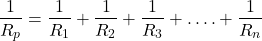where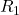,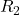, and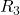are the resistance values of the individual resistors that are connected in parallel.

The total amount of current will often vary inversely with the level of cumulative resistance. There is a direct relation between the resistance of the individual resistors and the total resistance of the resistance collection.

If all endpoints of the resistors are wired to both endpoints of the power supply, so the resistors are connected in parallel and their equivalent resistance declines between their endpoints. There is more than one direction to flow in parallel circuit current.

To investigate this relationship, let’s start with the simplest case of two resistors positioned in parallel branches, each of which has the same resistance value as 4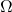. Since the circuit provides two equivalent paths for the charge transport, just one-half of the charge can choose to travel through the branch.

Although each branch gives 4of resistance to any charge flowing through it, only one-half of all the charge flowing through the circuit may meet 4of resistance of that branch. Thus, the presence of two 4resistors in parallel will be equal to one 2resistor in the circuit. This is the concept of equivalent resistance in a parallel circuit.

### Equivalent Resistance Series Circuit

If all components are connected in series, the circuit is referred to as a series circuit. In a series circuit, each unit is connected in such a way that there is only one route through which the charge can travel through the external circuit. Every charge traveling through the external circuit loop would travel through each resistor in a sequential manner. In a series circuit, the current has only one path to flow.

Charge flows together over the external circuit at a rate that is the same everywhere. The current is not stronger at one place and weaker at another point. Inversely, the exact amount of current varies with the total resistance. There is a direct relationship between the resistance of the single resistors and the total resistance of all the resistors present in the circuit.

For example, when two 6-Ω resistors are connected in series, it would be equivalent to having one 12-Ω resistor in the circuit. This is the concept of equivalent resistance in a series circuit.

For series circuits, the equivalent resistance of a series circuit is given as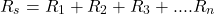If the endpoint of one resistor is linearly connected to the endpoint of the neighboring resistor and the free end of one resistor and the free end of the other resistor are connected to the power supply. Then the two resistors are wired in series and their equal resistance increases between their endpoints.

## Equivalent Resistance Examples

### For the Combination of Resistors Shown, Find the Equivalent Resistance Between Points A and B.

#### Example 1

For the given circuit below, what is the equivalent resistance between points A and B?

The two resistorsandwith value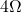are in series. So, their equivalent resistance value will be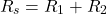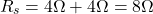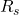,and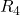are in parallel. The eqivalent resistance of the circuit.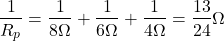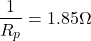#### Example 2

For the given circuit below, calculate the equivalent resistance between the end points A and B

The expression for the equivalent resistance of the resistor connected in series is given as follows.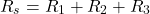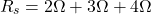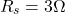### Which Circuit Has the Smallest Equivalent Resistance

#### Example 1

From the below given circuits, identify the circuit that has the smallest equivalent resistance.

The first given is a series circuit. So, the equivalent resistance is given as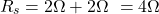The second given is a parallel circuit. So, the equivalent resistance is given as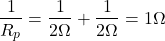The second given is also a parallel circuit. So, the equivalent resistance is given as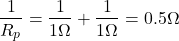The fourth given is a series circuit. So, the equivalent resistance is given as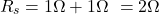So, from the above calculation it is seen that the third option has the smallest euqivalent resistance value.

### Difficult Equivalent Resistance Problems

#### Example 1

Find the Equivalent Resistance of the given circuit.

To get the Equivalent Resistance we combine resistors in series and in parallel. Here,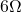and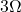are in parallel. So, the equivalent resistance is given as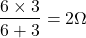Also, the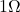and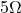resistors are in series. Hence the equivalent resistance will be given as,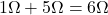After reduction, we now notice,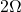andare in series, so the equivalent resistance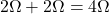Thisresistor is now in parallel with theresistor. So, their equivalent resistance will be given as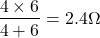Now replacing the above circuit with appropriate values, the three resistors will be in series. So, the final equivalent resistance is given as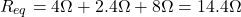#### Example 2

What is the equivalent resistance between points A and B?

To find the current through the battery we need to find the equivalent resistance of the circuit. The total current I is divided into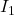and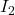. The currentpasses through tworesistors as they are connected in series and have the same current. The currentpasses throughand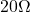resistors as they have the same current.

We need to find the currentby first calculating the current I that passes through the battery.

We see thatandresistors are connected in series. We replace them with an equivalent resistor with a resistance of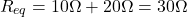Tworesistors are connected in series. We replace them with an equivalent resistance of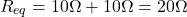Now we have two resistors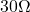andconnected in parallel. We can replace with an equivalent resistor.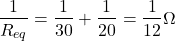Finally, we have two resistorsand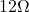connected in series. The equivalent resistance of these two resistors is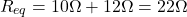Now we can find the current I through the battery. It is,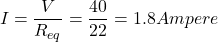This current is divided between two currentsand. So, the total current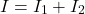(1)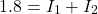The second equation, which relates the currents, is the condition that the voltage across the resistoris equal to the voltage across the resistor.

(2)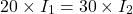From the above equations ((1) and (2) the currentis found.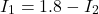Then we substitute this relation into equation (2),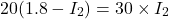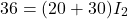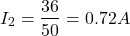So, now the current I_1 is given as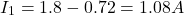Want To Learn Faster? 🎓
Get electrical articles delivered to your inbox every week.
No credit card required—it’s 100% free.